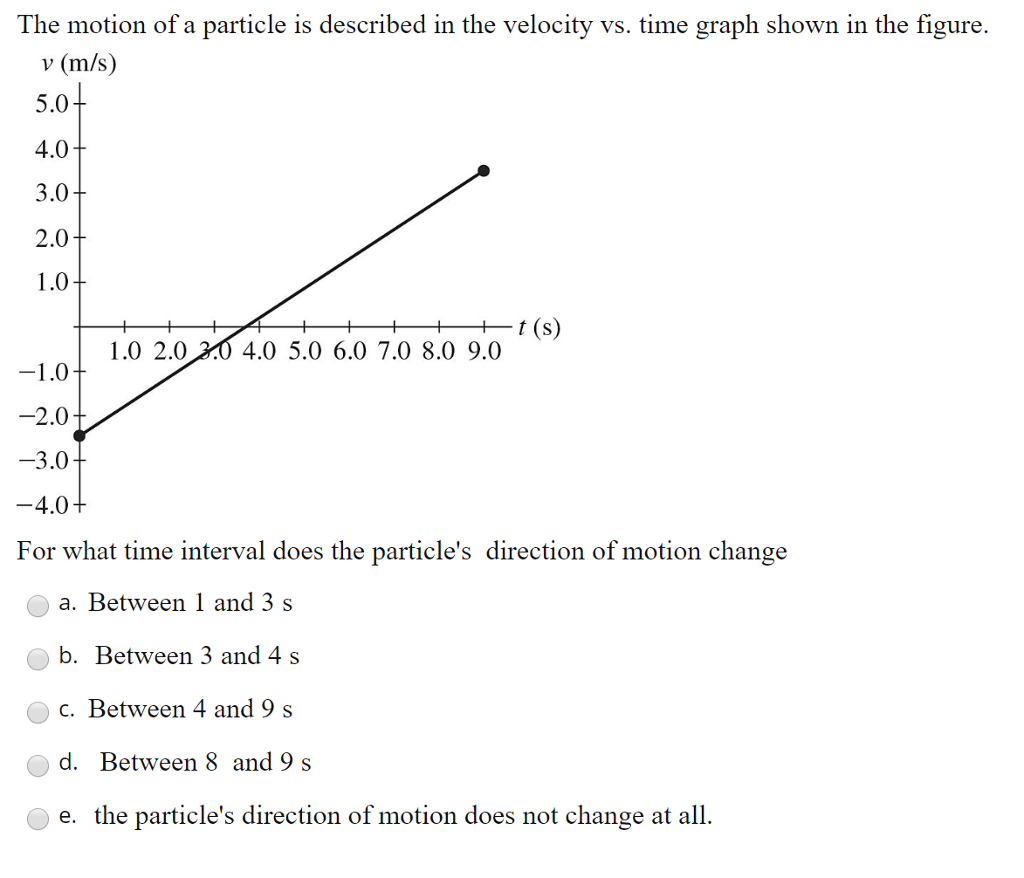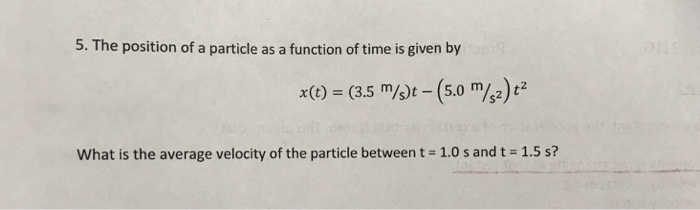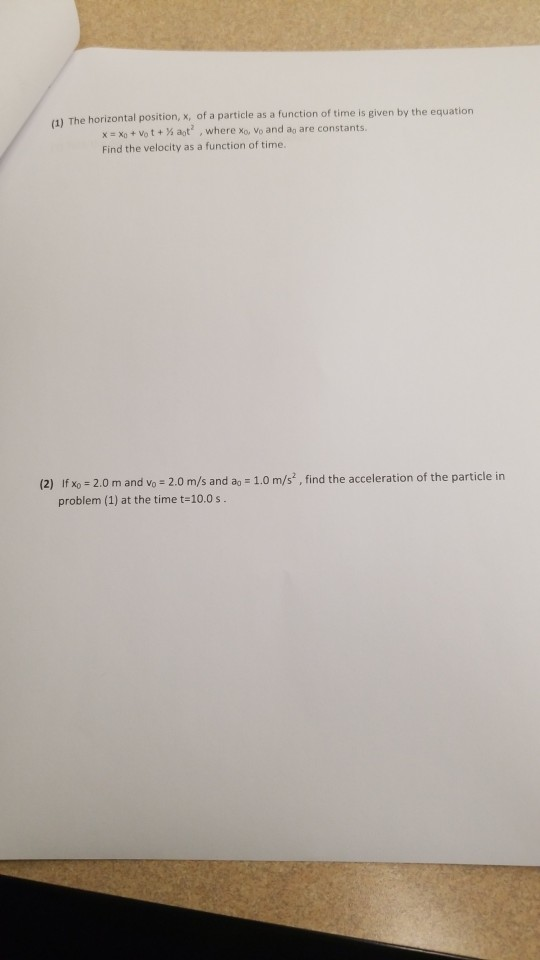Question

# The position of a particle is described by the function x = 3.0 t2 + 5.0...

`The position of a particle is described by the function x = 3.0 t2 + 5.0 t + 3.0 (m). Determine the average velocity (in m / s) for the time interval t1 = 2.0 s and t2 = 4.0 s.`

here,

x = 3 t^2 + 5 t + 3

at t1 = 2 s

x1 = 3 * (2)^2 + 5 * (2) + 3 m

x1 = 25 m

at t2 = 4 s

x2 = 3 * (4)^2 + 5 * (4) + 3 m

x2 = 71 m

the average velocity , v = ( x2 - x1)/(t2 - t1)

v = ( 71 - 25) m /(4 - 2) s

v = 23 m/s

#### Earn Coins

Coins can be redeemed for fabulous gifts.

Similar Homework Help Questions
• ### A particle at t1 = -2.0 s is at x1 = 5.0 cm and at t2...

A particle at t1 = -2.0 s is at x1 = 5.0 cm and at t2 = 5.0 s is at x2 = 8.9 cm . What is its average velocity over this time interval? Can you calculate its average speed from these data?

• ### The position of a particle for t > 0 is given by ?⃗(?) = (3.0 ?...

The position of a particle for t > 0 is given by ?⃗(?) = (3.0 ? ?̂ − 7.0 ? ?̂ − 5.0 ? ?) m (a) What is the velocity as a function of time? (b) What is the acceleration as a function of time? (c) What is the particle’s velocity at t = 2.0 s? (d) What is its speed at t = 1.0 s and t = 3.0 s? (e) What is the average velocity between t...

• ### The position of a particle moving along the x axis is given by x = (21 + 22t - 6.0t2) m

The position of a particle moving along the x axis is given by x = (21 + 22t - 6.0t2) m, where t is in s. What is the average velocity during the time interval t = 1.0 s to t = 3.0 s?-6.0 m/s-4.0 m/s -2.0 m/s-8.0 m/s8.0 m/s

• ### The position of a particle moving along the x axis is given by (21 22 6...

The position of a particle moving along the x axis is given by (21 22 6 0 )m 2 x = + t − . t , where t is in s. What is the average velocity during the time interval t = 1.0 s to t = 3.0 s? a) –6.0 m/s b) –4.0 m/s c) –2.0 m/s d) –8.0 m/s e) 8.0 m/s

• ### The motion of a particle is described in the velocity vs. time graph shown in the...The motion of a particle is described in the velocity vs. time graph shown in the figure. v (m/s) 5.0 4.0 3.0 2.0 1.0 2.02.0 4.0 5.0 6.0 7.0 8.0 9.0 2.0 3.0 4.0 For what time interval does the particle's direction of motion change a. Between 1 and 3 s b. Between 3 and 4 s c. Between 4 and 9 s d. Between 8 and 9 s e. the particle's direction of motion does not change at all

• ### 5. The position of a particle as a function of time is given by x(.5 m/)t...5. The position of a particle as a function of time is given by x(.5 m/)t -(5.0 m/z)2 what is the average velocity of the particle between t = 1.0 s and 1.5 s?

• ### The position of a particle moving along the x axis depends on the time according to the equation x = ct2-bt3

The position of a particle moving along the x axis depends on the time according to the equation x = ct2-bt3, where x is in meters and t in seconds. What are the units of (a) constant c and (b) constant b? Let their numerical values be 3.0 and 2.0, respectively. (c) At what time does the particle reach its maximum positive x position? From t=0.0s to t=4.0 s, (d) what distance does the particle move and (e) what is...

• ### The position of a particle as a function of time is given by r(t)=(-3.0m/s)ti +(6.0m)j+[ 7.0m-(4.0m/s^3)t^3]k...

The position of a particle as a function of time is given by r(t)=(-3.0m/s)ti +(6.0m)j+[ 7.0m-(4.0m/s^3)t^3]k a. what is the particle's displacement between t1=0 and t2=2.0s? b. determine the particle's instantaneous velocity as a function of time. c. what is the particle's average velocity between t1=0s and t2=2.0s? d. Is there a time when the particle has a velocity of zero? e. Determine the particle's instantaneous acceleration as a function of time? Can you please explain the formulas you used...

• ### The position of a particle moving along the x axis depends on the time according to...

The position of a particle moving along the x axis depends on the time according to the equation x = ct3 - bt7, where x is in meters and t in seconds. Let c and b have numerical values 2.5 m/s3 and 1.4 m/s7, respectively. From t = 0.0 s to t = 1.4 s, (a) what is the displacement of the particle? Find its velocity at times (b) 1.0 s, (c) 2.0 s, (d) 3.0 s, and (e) 4.0...

• ### horizontal position, x, of a particle as a function of time is given by the equation...horizontal position, x, of a particle as a function of time is given by the equation xo + vo t + ½ at' , where xa vo and ao are constants. Find the velocity as a function of time. (2) Ifx0 2.0 m and vo 2.0 m/s and ao 1.0 m/s, find the acceleration of the particle in problem (1) at the time t-10.0 s